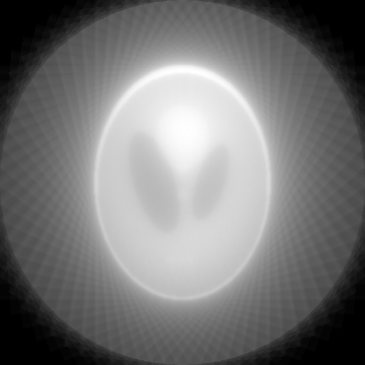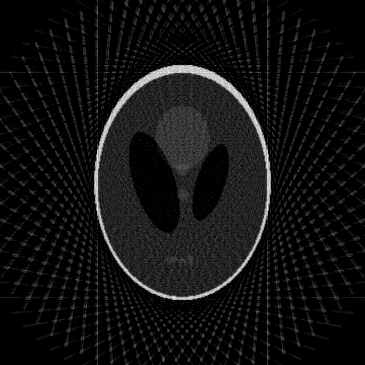Warning

## Lab 09 : CT Reconstruction, forward projection, backprojection

Topics – Math of CT images

• forward projection
• naive backprojection
• filtered backprojection

slides lab09_presentation.pdf

### HW09 Assignment

#### 1. Forward projection

The homework has four consecutive parts. When completed, you will have your own implementation of the forward projection ( Radon transform) used in CT imaging. A set of projections with varying direction $\theta$ is acquired. The object Cartesian coordinate system (grid) is denoted $x, y$. We shall also define a rotated coordinate system $p$, $q$, chosen so that projection corresponds to integration along the $p$ axis, creating a 1D function of $q$ for each angle $\theta$. Illustration of both coordinate systems is given below:

(Implementing our own Radon transform will help us to understand the inverse transform, which is the key concept for image reconstruction from measured CT projections. This will be the topic of the next lab.)

Exercise 1.1

The relationship between the two coordinate systems is given by \begin{align} p &=& x \cdot \cos \theta + y \cdot \sin \theta \\ q &=& -x \cdot \sin \theta + y \cdot \cos \theta \end{align} Find the smallest range $[-p_m, p_m] \times [-q_m, q_m]$ needed so that all points from $(x, y) \in [-x_m, x_m] \times [-y_m, y_m]$ fall into this range. Provide either a graphical or an analytical solution.

Exercise 1.2

Diskretize the Radon function \begin{equation} J(\theta,p) = \int_{-\infty}^\infty f\left ( p\cdot\cos(\theta) - q\cdot\sin(\theta) ~ , ~ p\cdot\sin(\theta) + q\cdot\cos(\theta) \right ) dq \end{equation} i.e. approximate the integral by a sum.

Homework 1.3 [1.5 pts]

Implement the function

function fwrad = myRadon(img, theta)
which will return the forward Radon transform fwrad of the input image img of dimension $[m \times n]$, given the array of projections angles theta of length $k$ (angles are provided in degrees). Size of the resulting sinogram fwrad is $[(2p_m + 1) \times k]$. Submit the code into BRUTE.

Homework 1.4 [0.5 pt]

Transform the Shepp-Logan phantom (matlab function phantom) of size 256 px for projection angles $\theta \in \{0^{\circ}, 1^{\circ}, 2^{\circ}, \ldots, 179^{\circ}\}$ with your function. Show the input and the resulting sinogram. Pay attention to labelling the axis correctly.

Hints

• set the image coordinate system such that $[x, y] = [0, 0]$ lies in the centre of the image, this leads to the spatial range $[-x_m, x_m] \times [-y_m, y_m]$
• compute the maximal values $p_m$ and $q_m$
• use the discrete form of (3) and compute $J(\theta, p_h)$
• think of points $p_h, q_h$ as of grid (meshgrid) rotated by (a fixed) $\theta$
• go over all $(p_h, q_h)$ on a grid, transforming each to a corresponding point $(x_h, y_h)$ in the input image
• as $(x_h, y_h)$ will in general not coincide with pixel centers, use interpolation ( interp2 function) to get $img(x_h, y_h)$
• compute the numerical value of $J(\theta, p_h)$ and store it into the fwrad sinogram.

#### 2. Backprojection

The main topic of the second part of today's lab is the inverse of the forward projection (Radon transform). The inverse transform starts with a sinogram, i.e. the projection image $J(\theta, p)$ and reconstructs the input image $f(x, y)$ in Cartesian space.

Naive approach The naive approach (the unfiltered back-projection) is just the reverse process of the projection – we smear the values of each projection back to the reconstructed image and add them together. We can write it as a sum over projection images $f_{\theta}(x, y)$ which we get from the measured projection $J(\theta, p)$ via $$f_{\theta}(x, y) = J(\theta, x \cos \theta + y \sin \theta)$$ The reconstructed image is then $$f_{\text{unfilt}}(x, y) = \mathcal{B}[\mathcal{R}f] = \sum_\theta {f_{\theta}(x, y)}$$

Optimal reconstruction From theoretical point of view, the central slice theorem gives us the optimal solution. The 2D-Fourier Transform $\mathcal{F}_2$ of the input image $f(x, y)$ is the same as the set of 1D-Fourier transformed projections $\mathcal{F}_1 [J(\theta, p)]$. However, since we have only very sparse samples in $\theta, p$, direct application of the inverse transform $\mathcal{F}_2^{-1}$ is impractical.

Nonetheless, we can use the central slice theorem to rewrite the Fourier equality $f(x, y) = \mathcal{F}_2^{-1} [\mathcal{F}_2 f]$ and with help of the Radon transform – $\mathcal{R}f$ to finally arrive at the formula:\begin{equation} f(x, y) = \mathcal{B} \{ \mathcal{F}_1^{-1} [|\omega| \cdot \mathcal{F}_1[\mathcal{R} f] \} \end{equation} i.e. the reconstructed image is obtained by back-projection $\mathcal{B}$ of the measured projections filtered by the ramp filter $|\omega|$ in Fourier domain.

Compared to the naive approach, the filtered back-projection computes $f_{\theta}$ from the filtered projection signal $J^{*}(\theta, p)$. To get $J^{*}(\theta, p)$ from the input projection data $J(\theta, p)$ we take a look at the inner part of equation (1) and write it only for a single projection:

$$\mathcal{B} \{ \mathcal{F}_1^{-1} [|\omega| \cdot \mathcal{F}_1[J(\theta, p)] \}$$

So we get either by multiplication in the Fourier domain or convolution in the spatial domain: $$J^{*}(\theta, p) = \mathcal{F}_1^{-1} [|\omega| \cdot \mathcal{F}_1[J(\theta, p)] = \mathcal{F}_1^{-1} [|\omega|] * J(\theta, p)$$

The ramp filter $|\omega|$ leads to optimal reconstruction, but when applied in Fourier-domain, it also acts like a high-pass filter (since unbounded on both ends) and is therefore amplifying noise frequencies. To minimize this effect, the filter is applied in combination with windows that reduce its frequency span. We will work with Hamming window (hamming) and the Shepp-Logan, also sinc window (shepp-logan). The last filter is ram-lak which does no windowing, but only cuts of high frequencies. Use the provided function H = designFilter(f_type, N, f_d) to get the frequency-domain filter - plot both $H$ and $\mathcal{F}_1^{-1}[H]$ to get better comprehension of the filtering functions.

Now we can summarize the (filtered) back-projection algorithm:

• compute the output image size
• initialize the target image $f(x, y) = 0$
• (get the filter H = designFilter(f_type, N, f_d) )
• for each projection angle $\theta$
• ( compute the filtered $J^{*}(\theta, p) = \mathcal{F}_1^{-1}[H * \mathcal{F}_1[J(\theta, p)]]$ or by convolution)
• ( compute the back-projection image $f_{\theta}(x, y)$ from $J*(\theta, p)$ )
• add the back-projection image $f_{\theta}(x, y)$ to $f(x, y)$
• normalize the reconstructed image by multiplying by $\frac{\pi}{2 \cdot n_\theta}$ (see equation 3.5.4 in https://web.eecs.umich.edu/~fessler/course/516/l/c-tomo.pdf)

Get the archive with input sinogram (noisy_radon.mat), reference image (phantom.mat) and the designFilter.m script.

Homework 2.1 [1.5 pts]

Implement the inverse Radon transform as a function with signature

function img = myIradon( projim, thetas, f_type, f_d)
where projim is the projection image of size $[m \times n_{\theta}]$ and theta is vector of length $n_{\theta}$ containing the projection angles. The last parameter f_type is optional and if set, it specifies the filtration to be applied during the back-projection, f_d is a parameter of the cut-off frequency of the filter f_type.

Homework 2.2 [1.0 pts]

Take the input radon image which was created with the set of projection angles ${0, 1, ..., 179^{\circ}}$ and reconstruct the original image with different back-projection setting. Visualize the reconstructed image along with the difference image to the provided phantom image for each of the 5 settings:

• unfiltered back-projection
• filtered back-projection with filters – ram-lak and hamming – and with filter cut-off frequencies – $d \in {0.7, 1.0}$
• for filtered back-projection: visualize the filter in the frequency and the spatial domain alongside with the reconstructed and error image

Homework 2.3 [0.5 pts]

Report the relative reconstruction error \begin{equation} R=\sqrt{ \frac{1}{\|x\| \cdot \|y\|} \cdot \frac{ \sum_{\forall x, \forall y}(\hat{f}(x,y)-f(x,y))^2}{\sum_{\forall x, \forall y} f(x,y)^2} } \end{equation} for reconstruction from previous subtask.

Homework BONUS [1 pt]

Select the best filter (with respect to the minimal reconstruction error) and reconstruct the image again, but use fewer projection angles (try with each 2nd, 5th and 10th angle), report the reconstructed images.

#### Implementation hints

• use the same meshgrid X, Y as last time for positioning the output image $f(x, y)$
• each time, we interpolate only the 1D signal ( $J(\theta_0, p)$ for a fixed $\theta_0$ ). With meshgrids P and[X, Y] reflecting the spatial relation between $p$ and $(x, y)$, computation of the back-projected $f_{\theta}$ can be done with interp1 function).
• the designFilter returns array of even length, if the radon image has odd length (in $p$), first enlarge $J(\theta_0, p)$ and then apply Fourier transform and the filter $H$.

#### How to check whether the results are correct

• for direct back projection you should
• be able recognize the Shepp-Logan phantom (the file phantom.mat) in the reconstructed image
• see the star artefact and blurring in the reconstructed image (star in case you use lower number of projection angles, with the full range of projection angles it rather bright ring or halo); the artefact is most clear when you don't crop the image to the appropriate dimensions• for filtered backprojection
• recognize the Shepp-Logan phantom (the file phantom.mat) in the reconstructed image
• in comparison to the direct back projection the artefacts should be less apparent• compare your results to the iradon function in Matlab (set parameter FILTER -  'none'  for direct back projection,  'ram-lak'  or other for filtered back projection)